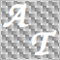• 概要
• レビュー (1)
• コメント

# MA AllTimeFrames

MA-AllTimeFrames shows six moving averages of M1, M5, M15, H1, H4, D1 timeframes in the timeframe of the chart.

All moving averages have the same period length and are calculated on the same price type.

### Parameters

• MaPeriod - the number of bars used for the indicator calculations. Proper values - from 5 to 250.
• MaMode
• SMA - Simple averaging
• EMA - Exponential averaging
• SMMA - Smoothed averaging
• LWMA - Linear-weighted averaging
• AppliedPrice
• CLOSE - Close price
• OPEN - Open price
• HIGH - The maximum price for the period
• LOW - The minimum price for the period
• MEDIAN - Median price, (high + low)/2
• TYPICAL - Typical price, (high + low + close)/3
• WEIGHTED - Average price, (high + low + close + close)/4

It is a simple indicator which can be quite useful if you quite often change the time period of your chart as the calculation of the moving averages is independent of that timeframe.

A lot of traders for expamle look at 20-period moving averages of their trading timeframe. So, you will quite often see bouncing at that displayed line.

Tip: If you drop one indicator working on the low price and one indicator working on the high price, you can see the possible bouncing ranges of the higher timeframes (H1, H4, D1).21376
2016.08.31 17:04

ユーザーはレーティングに対して何もコメントを残しませんでした